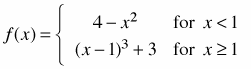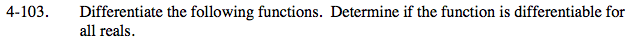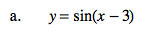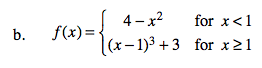### Home > CALC > Chapter 4 > Lesson 4.3.1 > Problem4-103

4-103.
1. Differentiate the following functions. Determine if the function is differentiable for all reals. Homework Help ✎

1. y = sin(x − 3)

2.Compare the graph of y = sin(x − 3) with its parent y = sinx.

y = sin(x − 3) looks just like y = sinx. Their periods are the same. Their amplitudes are the same. Their maximum and minimum y-values are the same. Their SLOPES are the same. The only difference is their horizontal locations.

The slopes will shift with the graph:
If y = sinxy = sin(x+3)
Then y' = cosxy' = cos(x + 3)The derivative will be a piecewise function as well. Differentiate each piece separately.

Consider the boundary point of the derivative. Examine the two pieces at x = 1. Is the derivative continuous?